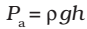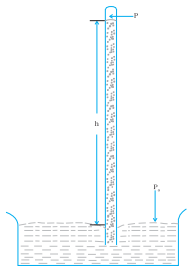The pressure of the atmosphere at any point is equal to the weight of a column of air of unit cross sectional area extending from that point to the top of the atmosphere.

A long glass tube closed at one end and filled with mercury is inserted into a trough of mercury as shown in Fig.(a) . This device is known as mercury barometer. The space above the mercury column in the tube contains only mercury vapor whose pressure P is so small that it may be neglected. The pressure inside the column at point A must equal the pressure at point B, which is at the same level. Pressure at B = atmospheric pressure = PaFig.(a). The mercury barometer

An open-tube manometer is a useful instrument for measuring pressure differences. It consists of a U-tube containing a suitable liquid i.e. a low-density liquid (such as oil) for measuring small pressure differences and a high-density liquid (such as mercury) for large pressure differences. One end of the tube is open to the atmosphere and another end is connected to the system whose pressure we want to measure [see Fig.(b)]. The pressure P at A is equal to the pressure at point B.Fig.(b). The open tube manometer

### Short but important points about Atmospheric pressure:

• It is about 100000 N/m2.
• It is equivalent to a weight of 10 tonnes on 1 m2.
• At sea level, atmospheric pressure is equal to 76 cm of the mercury column. Then, atmospheric pressure = h d g = 76 x 13.6 x 980 dyne/cm2 [The atmospheric pressure does not crush our body because the pressure of the blood flowing through our circulatory system] balanced this pressure.]
• Atmospheric pressure is also measured in torr and bar.
• 1 torr = 1 mm of mercury column
• 1 bar = l05 Pa
• The aneroid barometer is used to measure atmospheric pressure.

Click on a star to rate it!

Average rating 0 / 5. Vote count: 0

No votes so far! Be the first to rate this post.

As you found this post useful...